This book is archived and will be removed July 6, 2022. Please use the updated version.

Electromagnetic Waves

# 109 Energy Carried by Electromagnetic Waves

### Learning Objectives

By the end of this section, you will be able to:

• Express the time-averaged energy density of electromagnetic waves in terms of their electric and magnetic field amplitudes
• Calculate the Poynting vector and the energy intensity of electromagnetic waves
• Explain how the energy of an electromagnetic wave depends on its amplitude, whereas the energy of a photon is proportional to its frequency

Anyone who has used a microwave oven knows there is energy in electromagnetic waves. Sometimes this energy is obvious, such as in the warmth of the summer Sun. Other times, it is subtle, such as the unfelt energy of gamma rays, which can destroy living cells.

Electromagnetic waves bring energy into a system by virtue of their electric and magnetic fields. These fields can exert forces and move charges in the system and, thus, do work on them. However, there is energy in an electromagnetic wave itself, whether it is absorbed or not. Once created, the fields carry energy away from a source. If some energy is later absorbed, the field strengths are diminished and anything left travels on.

Clearly, the larger the strength of the electric and magnetic fields, the more work they can do and the greater the energy the electromagnetic wave carries. In electromagnetic waves, the amplitude is the maximum field strength of the electric and magnetic fields ((Figure)). The wave energy is determined by the wave amplitude.

Energy carried by a wave depends on its amplitude. With electromagnetic waves, doubling the E fields and B fields quadruples the energy density u and the energy flux uc.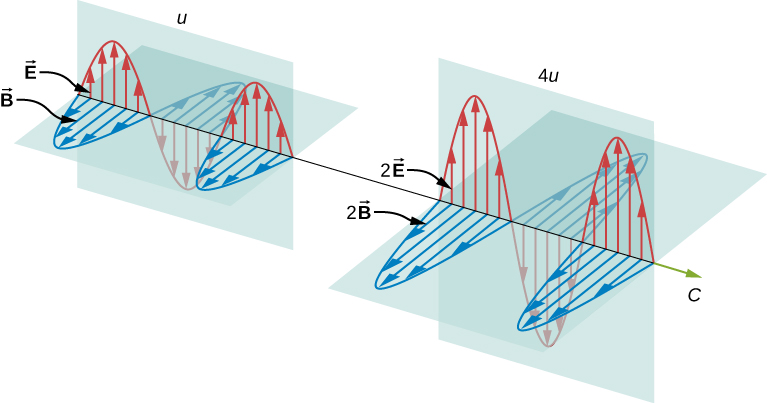For a plane wave traveling in the direction of the positive x-axis with the phase of the wave chosen so that the wave maximum is at the origin at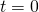, the electric and magnetic fields obey the equations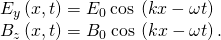The energy in any part of the electromagnetic wave is the sum of the energies of the electric and magnetic fields. This energy per unit volume, or energy density u, is the sum of the energy density from the electric field and the energy density from the magnetic field. Expressions for both field energy densities were discussed earlier (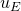in Capacitance and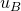in Inductance). Combining these the contributions, we obtain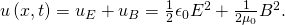The expression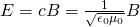then shows that the magnetic energy densityand electric energy densityare equal, despite the fact that changing electric fields generally produce only small magnetic fields. The equality of the electric and magnetic energy densities leads to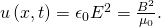The energy density moves with the electric and magnetic fields in a similar manner to the waves themselves.

We can find the rate of transport of energy by considering a small time interval. As shown in (Figure), the energy contained in a cylinder of length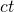and cross-sectional area A passes through the cross-sectional plane in the interval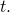The energy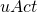contained in the electric and magnetic fields of the electromagnetic wave in the volume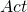passes through the area A in time.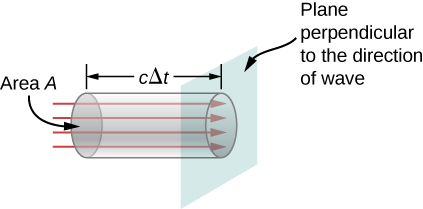The energy passing through area A in timeis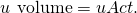The energy per unit area per unit time passing through a plane perpendicular to the wave, called the energy flux and denoted by S, can be calculated by dividing the energy by the area A and the time interval.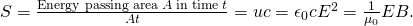More generally, the flux of energy through any surface also depends on the orientation of the surface. To take the direction into account, we introduce a vector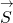, called the Poynting vector, with the following definition: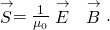The cross-product of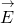and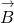points in the direction perpendicular to both vectors. To confirm that the direction ofis that of wave propagation, and not its negative, return to (Figure). Note that Lenz’s and Faraday’s laws imply that when the magnetic field shown is increasing in time, the electric field is greater at x than at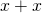. The electric field is decreasing with increasing x at the given time and location. The proportionality between electric and magnetic fields requires the electric field to increase in time along with the magnetic field. This is possible only if the wave is propagating to the right in the diagram, in which case, the relative orientations show that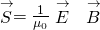is specifically in the direction of propagation of the electromagnetic wave.

The energy flux at any place also varies in time, as can be seen by substituting u from (Figure) into (Figure).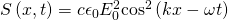Because the frequency of visible light is very high, of the order of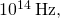the energy flux for visible light through any area is an extremely rapidly varying quantity. Most measuring devices, including our eyes, detect only an average over many cycles. The time average of the energy flux is the intensity I of the electromagnetic wave and is the power per unit area. It can be expressed by averaging the cosine function in (Figure) over one complete cycle, which is the same as time-averaging over many cycles (here, T is one period):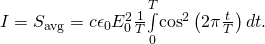We can either evaluate the integral, or else note that because the sine and cosine differ merely in phase, the average over a complete cycle for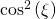is the same as for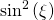, to obtain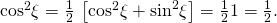where the angle bracketsstand for the time-averaging operation. The intensity of light moving at speed c in vacuum is then found to be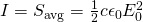in terms of the maximum electric field strength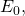which is also the electric field amplitude. Algebraic manipulation produces the relationship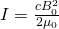where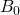is the magnetic field amplitude, which is the same as the maximum magnetic field strength. One more expression for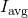in terms of both electric and magnetic field strengths is useful. Substituting the fact that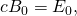the previous expression becomes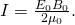We can use whichever of the three preceding equations is most convenient, because the three equations are really just different versions of the same result: The energy in a wave is related to amplitude squared. Furthermore, because these equations are based on the assumption that the electromagnetic waves are sinusoidal, the peak intensity is twice the average intensity; that is,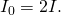A Laser Beam The beam from a small laboratory laser typically has an intensity of about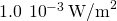. Assuming that the beam is composed of plane waves, calculate the amplitudes of the electric and magnetic fields in the beam.

Strategy Use the equation expressing intensity in terms of electric field to calculate the electric field from the intensity.

Solution From (Figure), the intensity of the laser beam is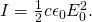The amplitude of the electric field is therefore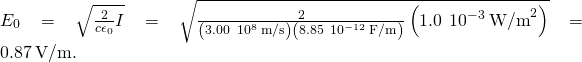The amplitude of the magnetic field can be obtained from (Figure):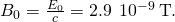Light Bulb Fields A light bulb emits 5.00 W of power as visible light. What are the average electric and magnetic fields from the light at a distance of 3.0 m?

Strategy Assume the bulb’s power output P is distributed uniformly over a sphere of radius 3.0 m to calculate the intensity, and from it, the electric field.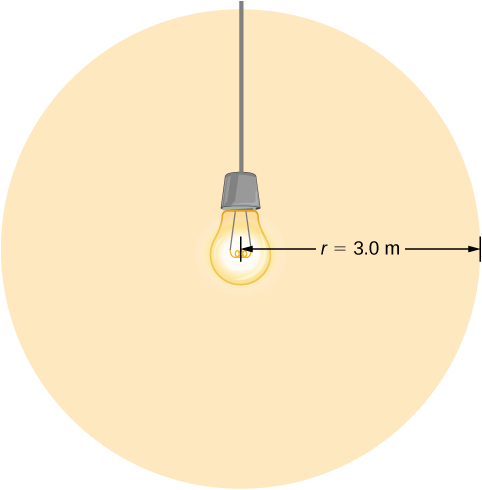Solution The power radiated as visible light is then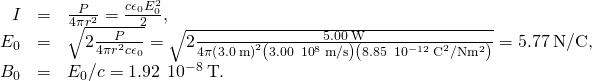Significance The intensity I falls off as the distance squared if the radiation is dispersed uniformly in all directions.

Radio Range A 60-kW radio transmitter on Earth sends its signal to a satellite 100 km away ((Figure)). At what distance in the same direction would the signal have the same maximum field strength if the transmitter’s output power were increased to 90 kW?

In three dimensions, a signal spreads over a solid angle as it travels outward from its source.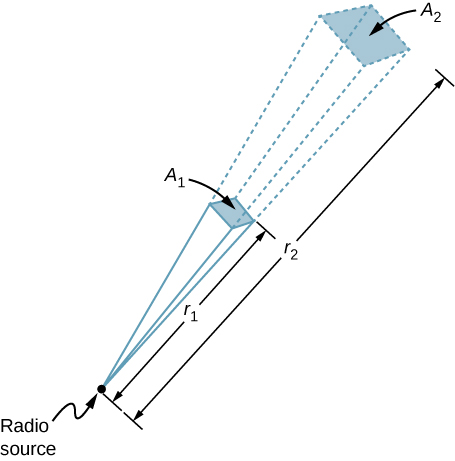Strategy The area over which the power in a particular direction is dispersed increases as distance squared, as illustrated in the figure. Change the power output P by a factor of (90 kW/60 kW) and change the area by the same factor to keep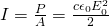the same. Then use the proportion of area A in the diagram to distance squared to find the distance that produces the calculated change in area.

Solution Using the proportionality of the areas to the squares of the distances, and solving, we obtain from the diagram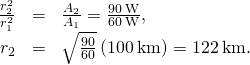Significance The range of a radio signal is the maximum distance between the transmitter and receiver that allows for normal operation. In the absence of complications such as reflections from obstacles, the intensity follows an inverse square law, and doubling the range would require multiplying the power by four.

### Summary

• The energy carried by any wave is proportional to its amplitude squared. For electromagnetic waves, this means intensity can be expressed as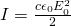where I is the average intensity in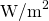and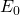is the maximum electric field strength of a continuous sinusoidal wave. This can also be expressed in terms of the maximum magnetic field strengthasand in terms of both electric and magnetic fields asThe three expressions forare all equivalent.

### Conceptual Questions

When you stand outdoors in the sunlight, why can you feel the energy that the sunlight carries, but not the momentum it carries?

The amount of energy (about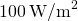) is can quickly produce a considerable change in temperature, but the light pressure (about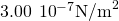) is much too small to notice.

How does the intensity of an electromagnetic wave depend on its electric field? How does it depend on its magnetic field?

What is the physical significance of the Poynting vector?

It has the magnitude of the energy flux and points in the direction of wave propagation. It gives the direction of energy flow and the amount of energy per area transported per second.

A 2.0-mW helium-neon laser transmits a continuous beam of red light of cross-sectional area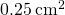. If the beam does not diverge appreciably, how would its rms electric field vary with distance from the laser? Explain.

### Problems

While outdoors on a sunny day, a student holds a large convex lens of radius 4.0 cm above a sheet of paper to produce a bright spot on the paper that is 1.0 cm in radius, rather than a sharp focus. By what factor is the electric field in the bright spot of light related to the electric field of sunlight leaving the side of the lens facing the paper?

A plane electromagnetic wave travels northward. At one instant, its electric field has a magnitude of 6.0 V/m and points eastward. What are the magnitude and direction of the magnetic field at this instant?

The magnetic field is downward, and it has magnitude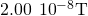.

The electric field of an electromagnetic wave is given by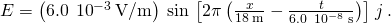Write the equations for the associated magnetic field and Poynting vector.

A radio station broadcasts at a frequency of 760 kHz. At a receiver some distance from the antenna, the maximum magnetic field of the electromagnetic wave detected is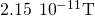.
(a) What is the maximum electric field? (b) What is the wavelength of the electromagnetic wave?

a.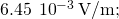b. 394 m

The filament in a clear incandescent light bulb radiates visible light at a power of 5.00 W. Model the glass part of the bulb as a sphere of radius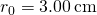and calculate the amount of electromagnetic energy from visible light inside the bulb.

At what distance does a 100-W lightbulb produce the same intensity of light as a 75-W lightbulb produces 10 m away? (Assume both have the same efficiency for converting electrical energy in the circuit into emitted electromagnetic energy.)

11.5 m

An incandescent light bulb emits only 2.6 W of its power as visible light. What is the rms electric field of the emitted light at a distance of 3.0 m from the bulb?

A 150-W lightbulb emits 5% of its energy as electromagnetic radiation. What is the magnitude of the average Poynting vector 10 m from the bulb?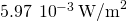A small helium-neon laser has a power output of 2.5 mW. What is the electromagnetic energy in a 1.0-m length of the beam?

At the top of Earth’s atmosphere, the time-averaged Poynting vector associated with sunlight has a magnitude of about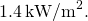(a) What are the maximum values of the electric and magnetic fields for a wave of this intensity? (b) What is the total power radiated by the sun? Assume that the Earth is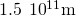from the Sun and that sunlight is composed of electromagnetic plane waves.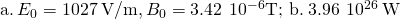The magnetic field of a plane electromagnetic wave moving along the z axis is given by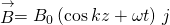, where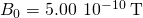and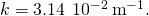(a) Write an expression for the electric field associated with the wave. (b) What are the frequency and the wavelength of the wave? (c) What is its average Poynting vector?

What is the intensity of an electromagnetic wave with a peak electric field strength of 125 V/m?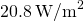Assume the helium-neon lasers commonly used in student physics laboratories have power outputs of 0.500 mW. (a) If such a laser beam is projected onto a circular spot 1.00 mm in diameter, what is its intensity? (b) Find the peak magnetic field strength. (c) Find the peak electric field strength.

An AM radio transmitter broadcasts 50.0 kW of power uniformly in all directions. (a) Assuming all of the radio waves that strike the ground are completely absorbed, and that there is no absorption by the atmosphere or other objects, what is the intensity 30.0 km away? (Hint: Half the power will be spread over the area of a hemisphere.) (b) What is the maximum electric field strength at this distance?

a.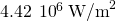; b.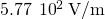Suppose the maximum safe intensity of microwaves for human exposure is taken to be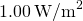. (a) If a radar unit leaks 10.0 W of microwaves (other than those sent by its antenna) uniformly in all directions, how far away must you be to be exposed to an intensity considered to be safe? Assume that the power spreads uniformly over the area of a sphere with no complications from absorption or reflection. (b) What is the maximum electric field strength at the safe intensity? (Note that early radar units leaked more than modern ones do. This caused identifiable health problems, such as cataracts, for people who worked near them.)

A 2.50-m-diameter university communications satellite dish receives TV signals that have a maximum electric field strength (for one channel) of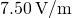(see below). (a) What is the intensity of this wave? (b) What is the power received by the antenna? (c) If the orbiting satellite broadcasts uniformly over an area of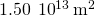(a large fraction of North America), how much power does it radiate?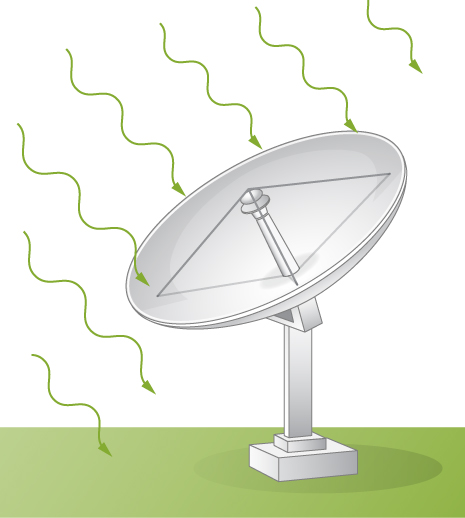a.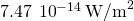; b.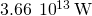; c. 1.12 W

Lasers can be constructed that produce an extremely high intensity electromagnetic wave for a brief time—called pulsed lasers. They are used to initiate nuclear fusion, for example. Such a laser may produce an electromagnetic wave with a maximum electric field strength of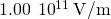for a time of 1.00 ns. (a) What is the maximum magnetic field strength in the wave? (b) What is the intensity of the beam? (c) What energy does it deliver on an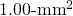area?

### Glossary

Poynting vector
vector equal to the cross product of the electric-and magnetic fields, that describes the flow of electromagnetic energy through a surface